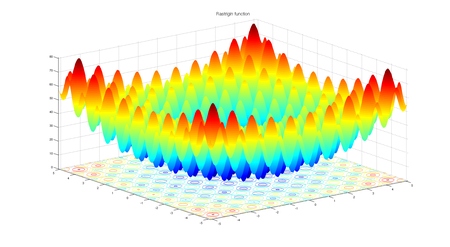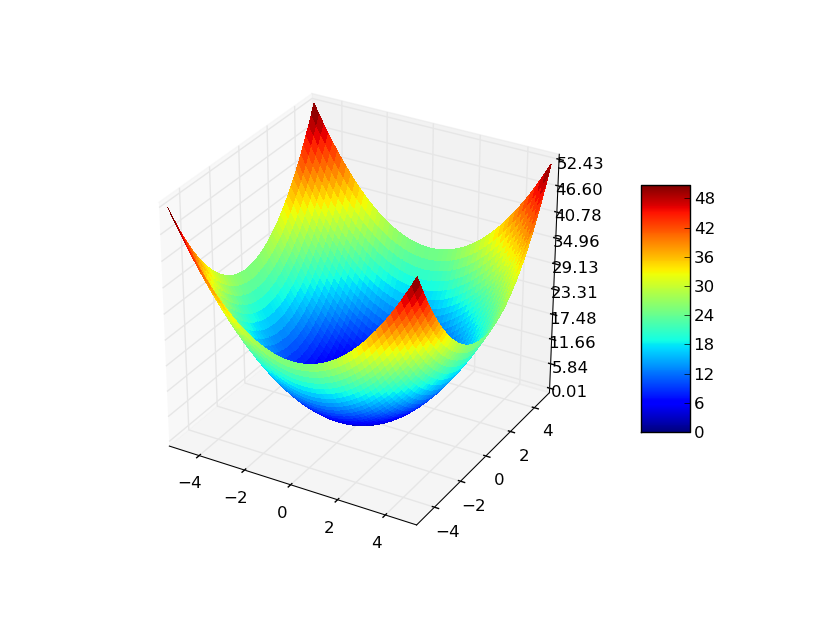# Implementation of Teaching Learning Based Optimization

• Last Updated : 10 Dec, 2021

The previous article Teaching Learning Based Optimization (TLBO) talked about the inspiration of teaching learning-based optimization, it’s mathematical modeling and algorithms. In this article we will implement Teaching learning-based optimization (TLBO) for two fitness functions 1) Rastrigin function    2) Sphere function.  The algorithm will run for a predefined number of maximum iterations and will try to find the minimum value of these fitness functions.

## Fitness function

### 1) Rastrigin function

Rastrigin function is a non-convex function and is often used as a performance test problem for optimization algorithms.

#### function equation:Fig1: Rastrigin function for 2 variables

For an optimization algorithm, rastrigin function is a very challenging one. Its complex behavior causes optimization algorithms to often be stuck at local minima. Having a lot of cosine oscillations on the plane introduces the complex behavior to this function.

### 2) Sphere function

Sphere function is a standard function for evaluating the performance of an optimization algorithm.

#### function equation:Fig 2: Sphere function for 2 variables

## Choice of hyper-parameters

### Parameters of problem:

• Number of dimensions (d) = 3
• Lower bound (minx) = -10.0
• Upper bound (maxx) = 10.0

### Hyperparameters of the algorithm:

• Number of particles (N) = 50
• Maximum number of iterations (max_iter) = 100

### Inputs

• Fitness function
• Problem parameters ( mentioned above)
• Population size (N) and Maximum number of iterations  (max_iter)
• Algorithm Specific hyperparameters (None in teaching-learning based optimization)

## Pseudocode

The pseudocode of the teaching-learning-based optimization is already described in the previous article. Data structures to store students as well as a data structure to store data specific to the individual students were also discussed.

## Python3

 # python implementation of Teaching learning based optimization (TLBO)# minimizing rastrigin and sphere function import randomimport math    # cos() for Rastriginimport copy    # array-copying convenienceimport sys     # max float  #-------fitness functions--------- # rastrigin functiondef fitness_rastrigin(position):  fitness_value = 0.0  for i in range(len(position)):    xi = position[i]    fitness_value += (xi * xi) - (10 * math.cos(2 * math.pi * xi)) + 10  return fitness_value #sphere functiondef fitness_sphere(position):    fitness_value = 0.0    for i in range(len(position)):        xi = position[i]        fitness_value += (xi*xi);    return fitness_value;#------------------------- #Student classclass Student:  def __init__(self, fitness, dim, minx, maxx, seed):    self.rnd = random.Random(seed)     # a list of size dim    # with 0.0 as value of all the elements    self.position = [0.0 for i in range(dim)]     # loop dim times and randomly select value of decision var    # value should be in between minx and maxx    for i in range(dim):      self.position[i] = ((maxx - minx) *        self.rnd.random() + minx)     # compute the fitness of student    self.fitness = fitness(self.position)  # Teaching learning based optimizationdef tlbo(fitness, max_iter, n, dim, minx, maxx):  rnd = random.Random(0)   # create n random students  classroom = [Student(fitness, dim, minx, maxx, i) for i in range(n)]   # compute the value of best_position and best_fitness in the classroom  Xbest = [0.0 for i in range(dim)]  Fbest = sys.float_info.max          for i in range(n): # check each Student    if classroom[i].fitness < Fbest:      Fbest = classroom[i].fitness      Xbest = copy.copy(classroom[i].position)   # main loop of tlbo  Iter = 0  while Iter < max_iter:         # after every 10 iterations    # print iteration number and best fitness value so far    if Iter % 10 == 0 and Iter > 1:      print("Iter = " + str(Iter) + " best fitness = %.3f" % Fbest)     # for each student of classroom    for i in range(n):       ### Teaching phase of ith student       # compute the mean of all the students in the class      Xmean = [0.0 for i in range(dim)]      for k in range(n):          for j in range(dim):              Xmean[j]+= classroom[k].position[j]             for j in range(dim):          Xmean[j]/= n;             # initialize new solution      Xnew = [0.0 for i in range(dim)]       # teaching factor (TF)      # either 1 or 2 ( randomly chosen)      TF = random.randint(1, 3)       # best student of the class is teacher      Xteacher = Xbest       # compute new solution      for j in range(dim):          Xnew[j] = classroom[i].position[j] + rnd.random()*(Xteacher[j] - TF*Xmean[j])             # if Xnew < minx OR Xnew > maxx      # then clip it      for j in range(dim):          Xnew[j] = max(Xnew[j], minx)          Xnew[j] = min(Xnew[j], maxx)             # compute fitness of new solution      fnew = fitness(Xnew)       # if new solution is better than old      # replace old with new solution      if(fnew < classroom[i].fitness):          classroom[i].position = Xnew          classroom[i].fitness = fnew              # update best student      if(fnew < Fbest):          Fbest = fnew          Xbest = Xnew         ### learning phase of ith student       # randomly choose a solution from classroom      # chosen solution should not be ith student      p = random.randint(0, n-1)      while(p==i):          p = random.randint(0, n-1)             # partner solution      Xpartner = classroom[p]       Xnew = [0.0 for i in range(dim)]      if(classroom[i].fitness < Xpartner.fitness):          for j in range(dim):              Xnew[j] = classroom[i].position[j] + rnd.random()*(classroom[i].position[j] - Xpartner.position[j])      else:          for j in range(dim):              Xnew[j] = classroom[i].position[j] - rnd.random()*(classroom[i].position[j] - Xpartner.position[j])       # if Xnew < minx OR Xnew > maxx      # then clip it      for j in range(dim):          Xnew[j] = max(Xnew[j], minx)          Xnew[j] = min(Xnew[j], maxx)             # compute fitness of new solution      fnew = fitness(Xnew)       # if new solution is better than old      # replace old with new solution      if(fnew < classroom[i].fitness):          classroom[i].position = Xnew          classroom[i].fitness = fnew              # update best student      if(fnew < Fbest):          Fbest = fnew          Xbest = Xnew     Iter += 1  # end-while   # return best student from classroom  return Xbest# end pso  #----------------------------# Driver code for rastrigin function print("\nBegin  teaching learning based optimization on rastrigin function\n")dim = 3fitness = fitness_rastrigin  print("Goal is to minimize Rastrigin's function in " + str(dim) + " variables")print("Function has known min = 0.0 at (", end="")for i in range(dim-1):  print("0, ", end="")print("0)") num_particles = 50max_iter = 100 print("Setting num_particles = " + str(num_particles))print("Setting max_iter    = " + str(max_iter))print("\nStarting TLBO algorithm\n")   best_position = tlbo(fitness, max_iter, num_particles, dim, -10.0, 10.0) print("\nTLBO completed\n")print("\nBest Student found:")print(["%.6f"%best_position[k] for k in range(dim)])fitness_value = fitness(best_position)print("fitness of best Student = %.6f" % fitness_value) print("\nEnd TLBO for rastrigin function\n")  print()print()  # Driver code for Sphere functionprint("\nBegin teaching learning based optimization on sphere function\n")dim = 3fitness = fitness_sphere  print("Goal is to minimize sphere function in " + str(dim) + " variables")print("Function has known min = 0.0 at (", end="")for i in range(dim-1):  print("0, ", end="")print("0)") num_particles = 50max_iter = 100 print("Setting num_particles = " + str(num_particles))print("Setting max_iter    = " + str(max_iter))print("\nStarting TLBO algorithm\n")   best_position = tlbo(fitness, max_iter, num_particles, dim, -10.0, 10.0) print("\nTLBO completed\n")print("\nBest Student found:")print(["%.6f"%best_position[k] for k in range(dim)])fitness_value = fitness(best_position)print("fitness of best Student = %.6f" % fitness_value) print("\nEnd TLBO for sphere function\n")

## Output

Begin  teaching learning based optimization on rastrigin function

Goal is to minimize Rastrigin's function in 3 variables
Function has known min = 0.0 at (0, 0, 0)
Setting num_particles = 50
Setting max_iter    = 100

Starting TLBO algorithm

Iter = 10 best fitness = 3.662
Iter = 20 best fitness = 0.389
Iter = 30 best fitness = 0.389
Iter = 40 best fitness = 0.389
Iter = 50 best fitness = 0.200
Iter = 60 best fitness = 0.132
Iter = 70 best fitness = 0.051
Iter = 80 best fitness = 0.003
Iter = 90 best fitness = 0.001

TLBO completed

Best Student found:
['0.000593', '-0.000040', '-0.000461']
fitness of best Student = 0.000112

End TLBO for rastrigin function

Begin teaching learning based optimization on sphere function

Goal is to minimize sphere function in 3 variables
Function has known min = 0.0 at (0, 0, 0)
Setting num_particles = 50
Setting max_iter    = 100

Starting TLBO algorithm

Iter = 10 best fitness = 0.009
Iter = 20 best fitness = 0.000
Iter = 30 best fitness = 0.000
Iter = 40 best fitness = 0.000
Iter = 50 best fitness = 0.000
Iter = 60 best fitness = 0.000
Iter = 70 best fitness = 0.000
Iter = 80 best fitness = 0.000
Iter = 90 best fitness = 0.000

TLBO completed

Best Student found:
['0.000000', '-0.000000', '-0.000000']
fitness of best Student = 0.000000

End TLBO for sphere function

### References

Research paper: R. V. Rao, V. J. Savsani & J. Balic (2012) Teaching–learning-based optimization algorithm for unconstrained and constrained real-parameter optimization problems, Engineering Optimization, 44:12, 1447-1462, DOI: 10.1080/0305215X.2011.652103

Inspiration of the implementation: https://www.mathworks.com/matlabcentral/fileexchange/65628-teaching-learning-based-optimization#:~:text=Teaching%20Learning%20Based%20Optimization%20is,teacher%20and%20the%20student%20phase.

My Personal Notes arrow_drop_up